# Fall Multiplication Puzzles

Looking for a fun way to practice multiplication this fall? Check out these FREE multiplication puzzles for some fall fun. These FREE Fall fun multiplication puzzles are easy to prep and learners can learn and have fun at the same time. Simple download the fall fun multiplication puzzles printables pdf file and great ready make learning multiplication for 2nd grade, 3rd grade, and 4th grade students FUN!## Fall Multiplication Puzzles – Free Printable

Scroll to the bottom of this post and enter in your details in the ORANGE box at the end of this post. You should receive an email with the printable. Do check your junk or spam email, as it might have ended up there as well. If you have any issues please contact me at thechattykinder@gmail.com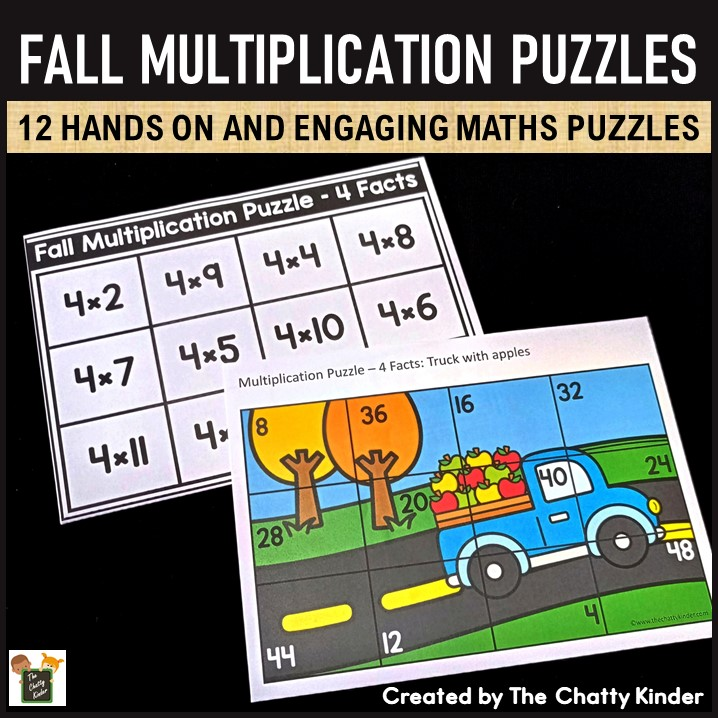## Teaching Multiplication

For me personally, I prefer teaching maths as a hands-on activity using manipulatives and visual aids making sure the learner understands the concept rather than just rote memorization of the facts.

When teaching Trucker his multiplication facts, I made sure we went through the basic concepts behind multiplication such as repeated addition, arrays and skip counting  before we revised the multiplication facts. We spent quite a number of days doing the multiplication fact booklets before I made him repeat any of his multiplication facts. This helped him to firm up his multiplication concepts and facts really well that he rarely refers to his multiplication posters.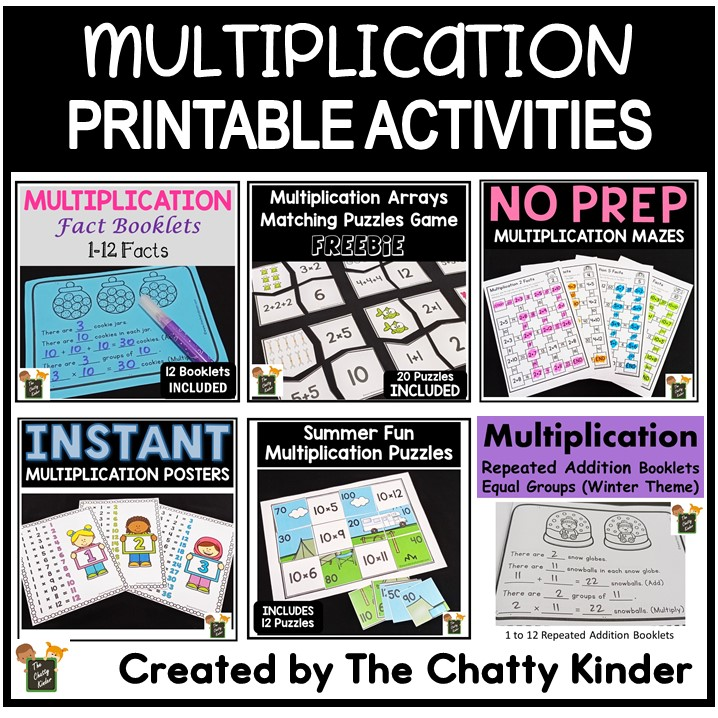## Multiplication Puzzles – A Fun and Easy Way to Practice Multiplication

Well, if you know me by now you might see that I enjoy creating puzzles. And this printable is just adding to the pile of puzzles. I create simple and low-prep puzzles that are easy to cut apart. I hate cutting the round corners in puzzles so I create these puzzles in a grid format. These puzzles are the same as the Summer Fun Multiplication Puzzles, except that these come in a Fall theme.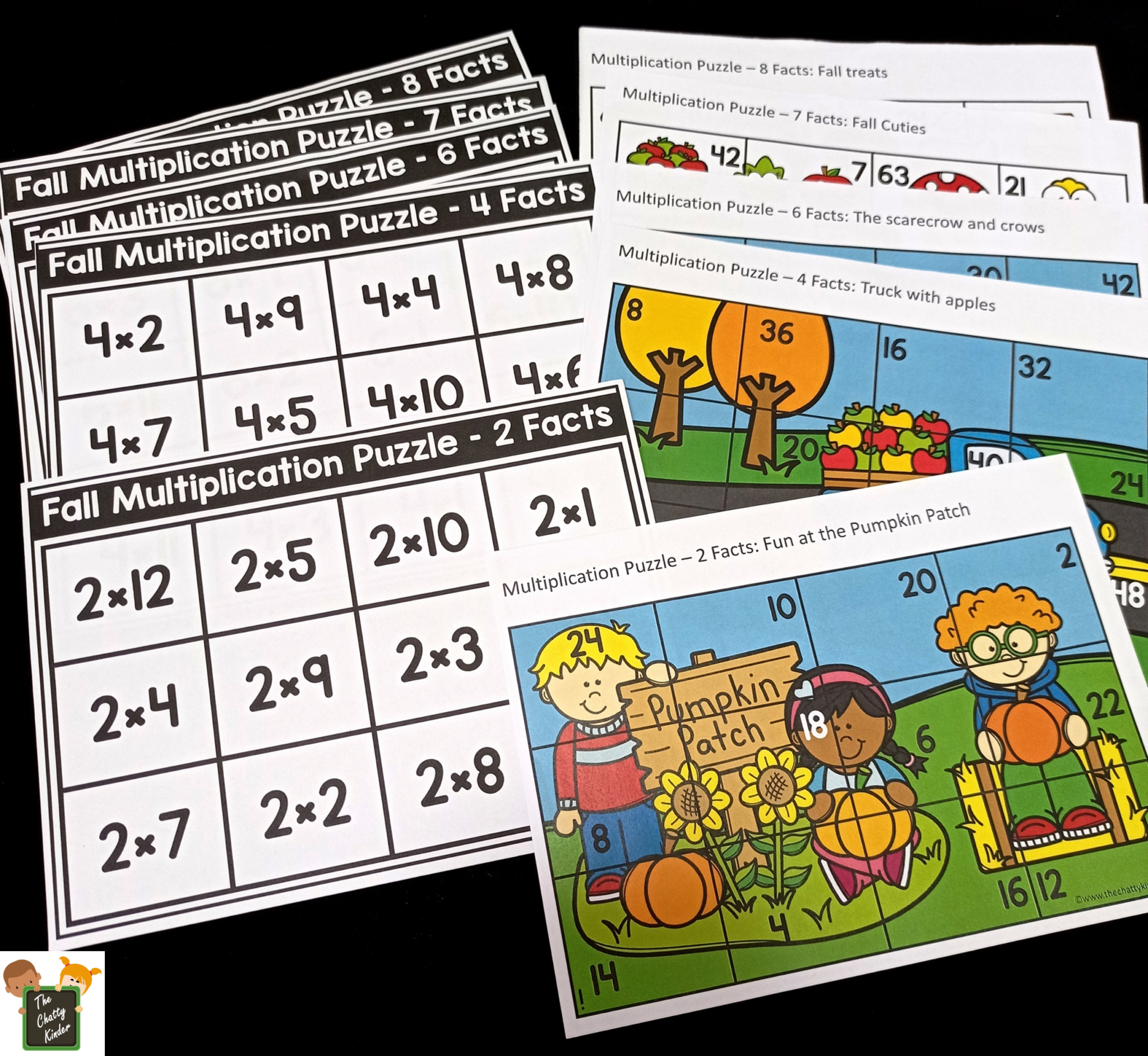In these multiplication puzzles learners will have to solve the multiplication equation on the grid. Then they will have to pick up the puzzle piece that contains that number of the solved multiplication fact and place it on that square. For e.g. if the multiplication in the square is 2×2, then the learner will have to know that the answer to this fact is 4, and thus will have to pick up the puzzle piece which has the number 4 on it and place it on to the multiplication equation of 2×2.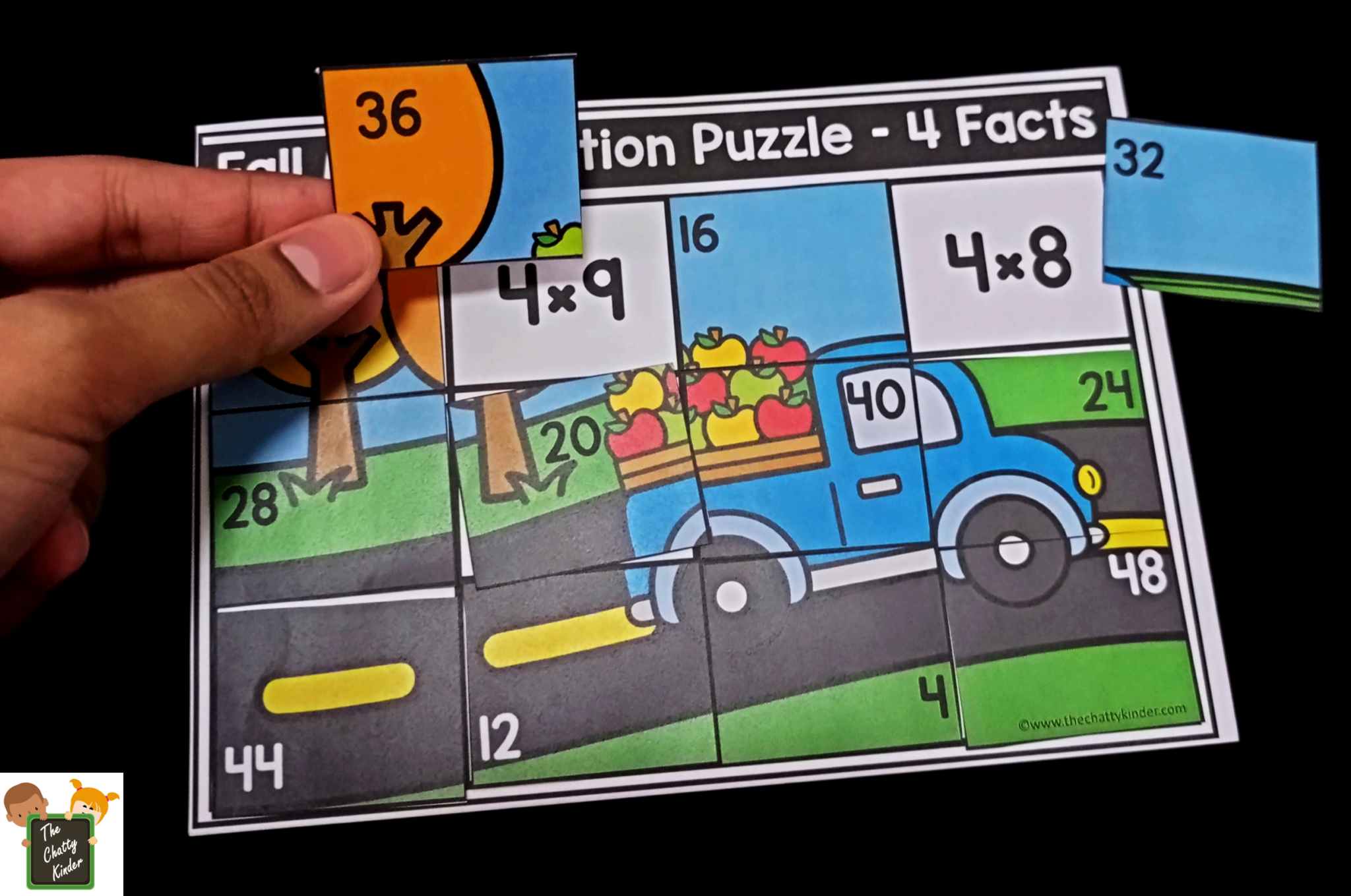This is an ideal hands-on practice to firm up the multiplication facts that the learner is already familiar with. The printable contains puzzles all the way from 1 to 12 Facts for a total of 12 puzzles.

## Using the Multiplication Puzzles:

These puzzles can be used during Fall for seasonal multiplication practice or can be used anytime of the year for practicing those tricky multiplication facts. If you need to differentiate this activity for your learners, simply choose different puzzle facts for your learner depending on which facts they are struggling or need additional practice on.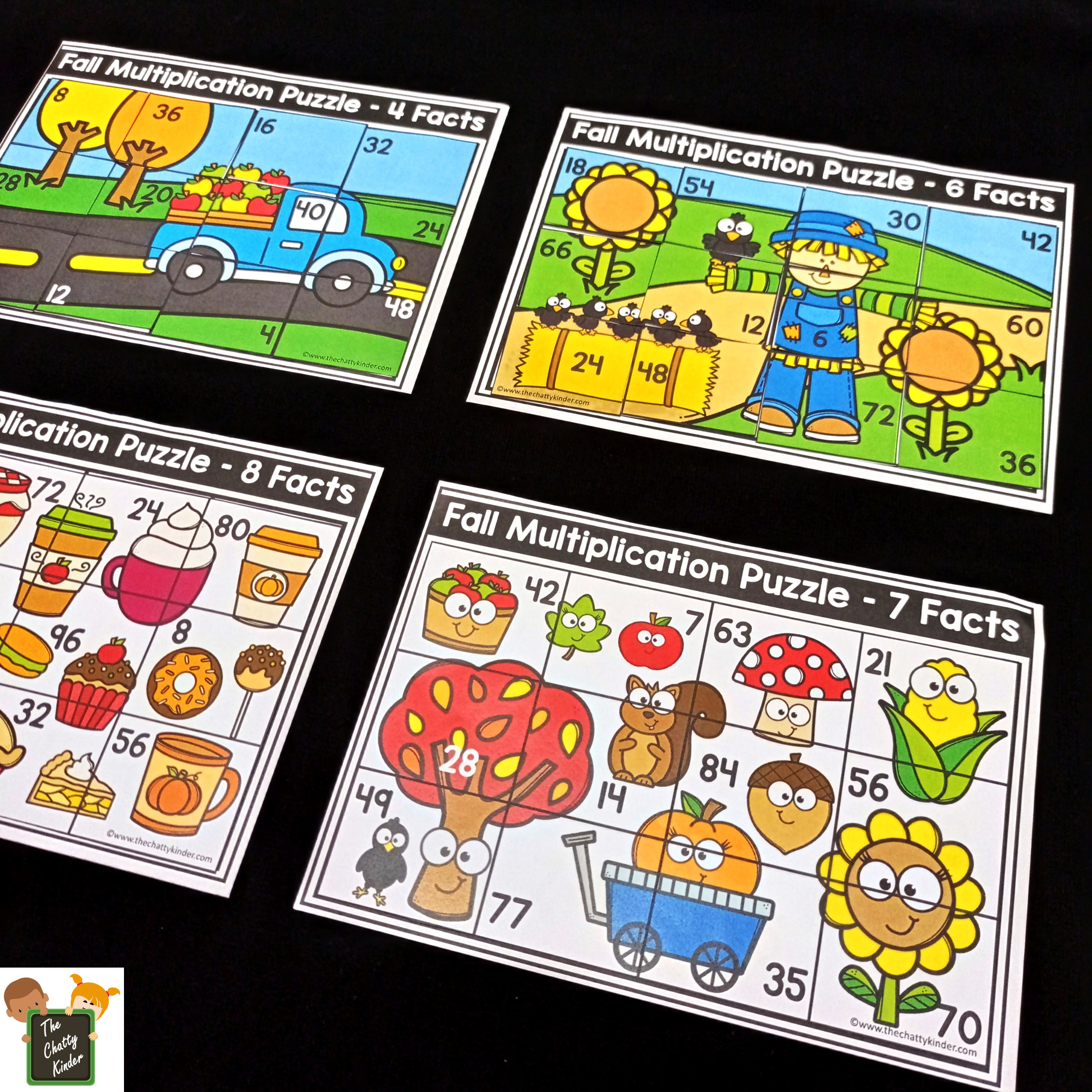### Materials Required:

• Printable (download the free printable at the end of this post)
• Printer
• Laminator (recommended)
• Paper cutter or scissors
• Laminating sheets

### To Prep:

• Download and print the pages you require.
• Laminate the pages and cut apart the puzzle pieces. Keep the puzzle mats as is.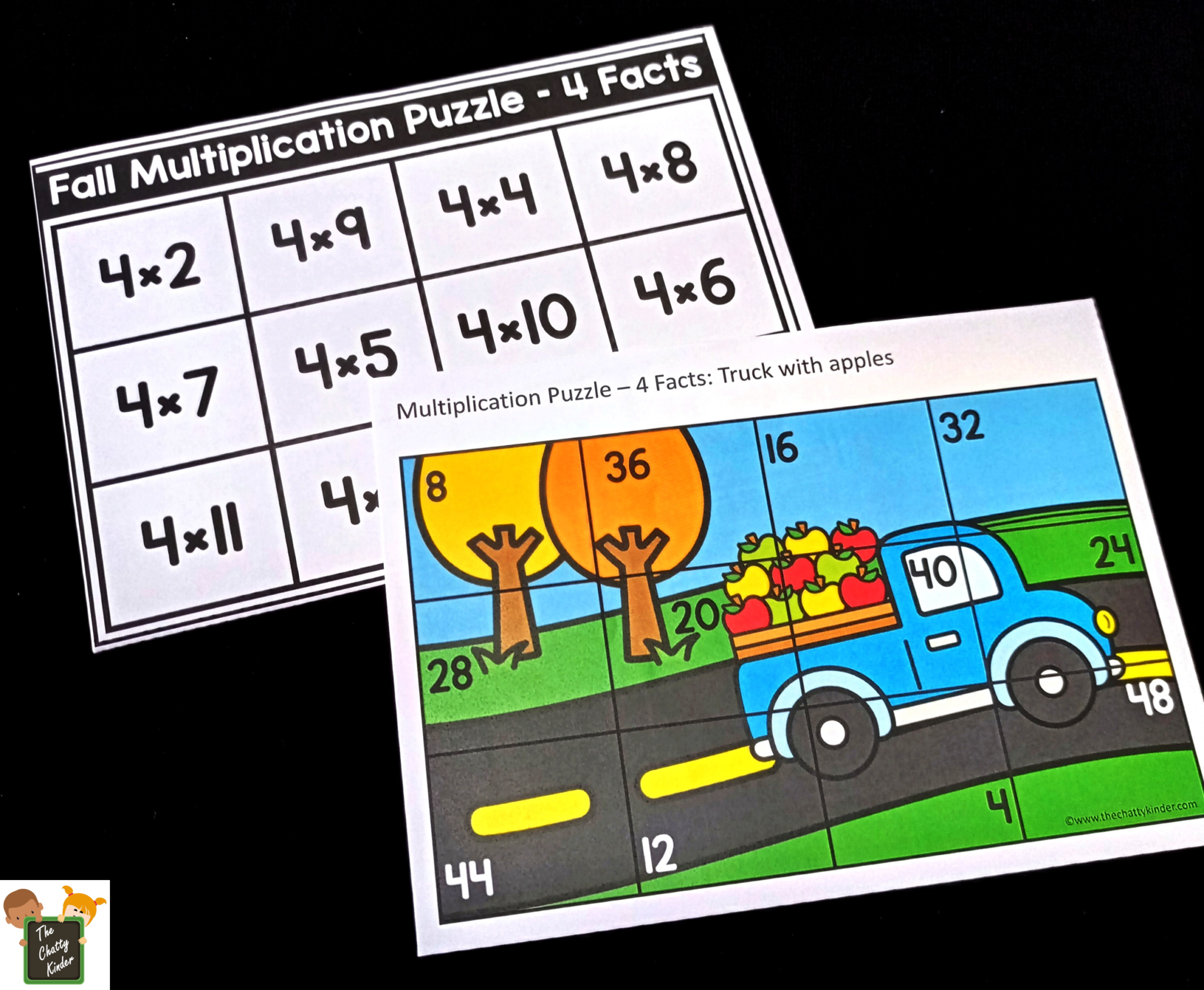### Presentation:

• Present the multiplication mat you’d like to review to your learner with the matching puzzle pieces.
• Before starting the puzzle, I would recommend you to revise the multiplication facts so students are familiar with their facts before they start on the puzzles.
• The learner will solve the equation in each grid, and find the puzzle piece with the matching number, and place the piece on top of the equation.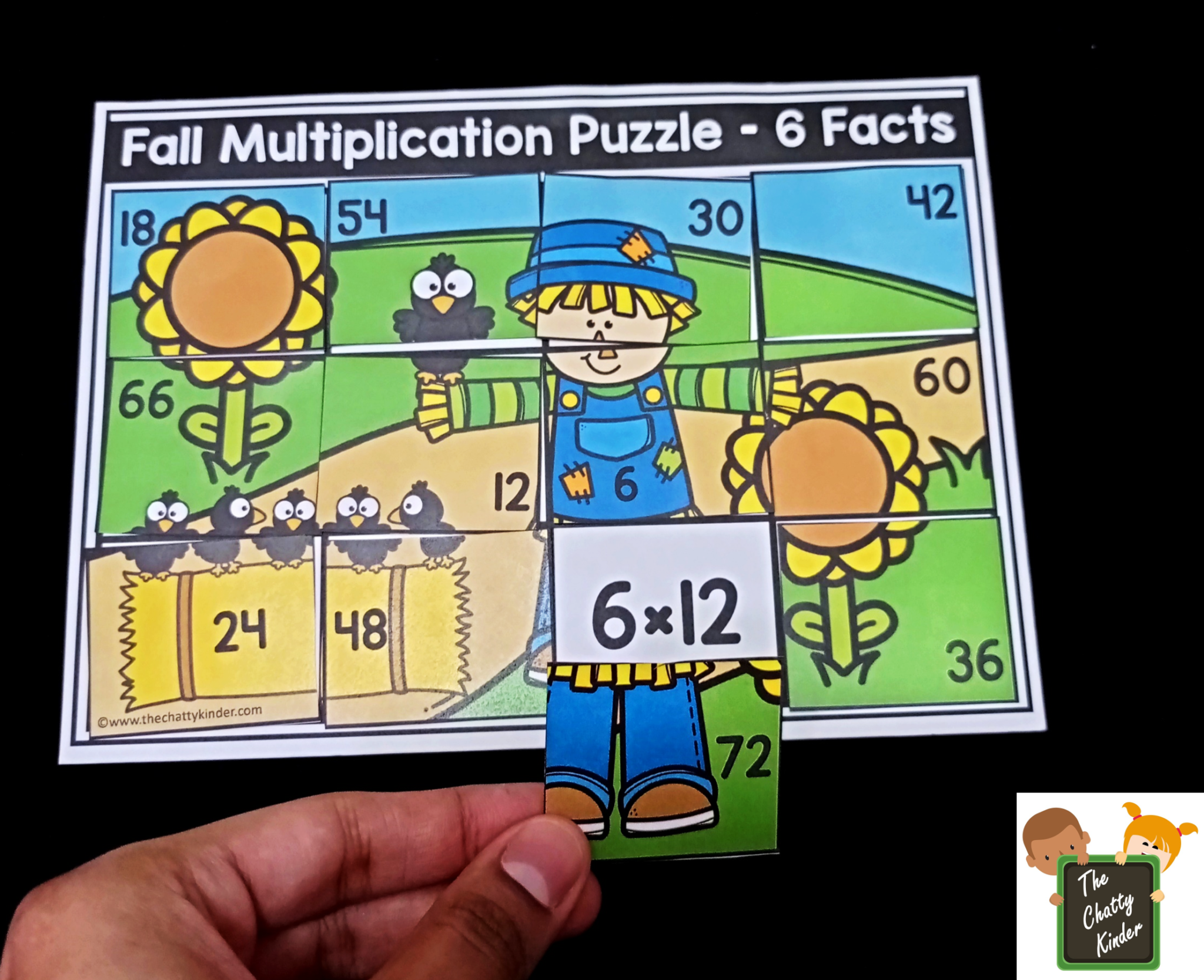• They will continue working their way through the grid until they have solved the puzzle completely.
• Learners will have so much fun working through the puzzle that they won’t even realize they are practicing their multiplication facts.This multiplication math activity, when done a few times will help to firm up the maths concepts and make practicing multiplication facts so much easier and fun.

Enter your details into the orange box at the end of this post to grab your FREE Fall Fun Multiplication Puzzles Printable! Enjoy!